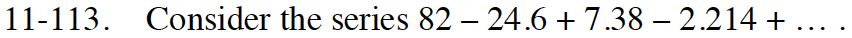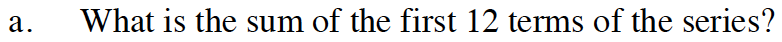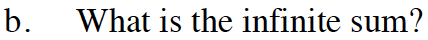Home > INT3 > Chapter 11 > Lesson 11.2.4 > Problem11-113

11-113.
1. Consider the series 82 – 24.6 + 7.38 – 2.214 + … . Homework Help ✎

1. What is the sum of the first 12 terms of the series?

2. What is the infinite sum?See the Math Notes box in Lesson 10.3.1 for the formulas for the sum of geometric series.$S=\frac{82}{1-(-0.3)}\approx 63.077$